# Work, Power, Potential Energy and Kinetic Energy Relations (Part - 2) Civil Engineering (CE) Notes | EduRev

## Civil Engineering (CE) : Work, Power, Potential Energy and Kinetic Energy Relations (Part - 2) Civil Engineering (CE) Notes | EduRev

The document Work, Power, Potential Energy and Kinetic Energy Relations (Part - 2) Civil Engineering (CE) Notes | EduRev is a part of the Civil Engineering (CE) Course Introduction to Dynamics and Vibrations- Notes, Videos, MCQs.
All you need of Civil Engineering (CE) at this link: Civil Engineering (CE)

Example 1: Potential energy of forces exerted by a spring. A free body diagram showing the forces exerted by a spring connecting two objects is shown in the figure.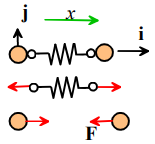1. The force exerted by a spring is F = -k(x- L0)i

2. The position vector of the force is r = xi
3. The potential energy follows as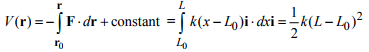where we have taken the constant to be zero.

Example 2: Potential energy of electrostatic forces exerted by charged particles. The figure shows two charged particles a distance x apart. To calculate the potential energy of the force acting on particle 2, we place particle 1 at the origin, and note that the force acting on particle 2 is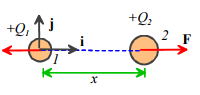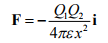where Q1 and Q2 are the charges on the two particles, and ε is a fundamental physical constant known as the Permittivity of the medium surrounding the particles. Since the force is zero when the particles are infinitely far apart, we take r0 at infinity. The potential energy follows as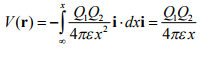Table of potential energy relations

In practice, however, we rarely need to do the integrals to calculate the potential energy of a force, because there are very few different kinds of force. For most engineering calculations the potential energy formulas listed in the table below are sufficient.

 Type of force Force vector Potential energy Gravity acting on a particle near earths surface F = -mgj V = mgy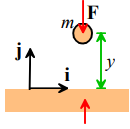Gravitational force exerted on mass m by mass M at the origin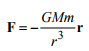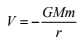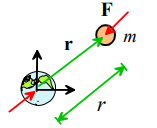Force exerted by a spring with stiffness k and unstretched length L0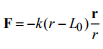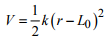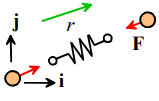Force acting between two charged particles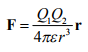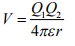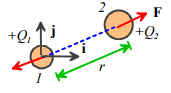Force exerted by one molecule of a noble gas (e.g. He, Ar, etc) on another (Lennard Jones potential). a is the equilibrium spacing between molecules, and E is the energy of the bond.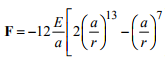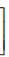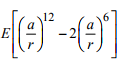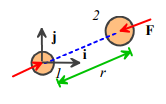4.1.5 Potential energy of concentrated moments exerted by a torsional spring

A potential energy cannot usually be defined for concentrated moments, because rotational motion is itself path dependent (the orientation of an object that is given two successive rotations depends on the order in which the rotations are applied). A potential energy can, however, be defined for the moments exerted by a torsional spring.A solid rod is a good example of a torsional spring. You could take hold of the ends of the rod and twist them, causing one end to rotate relative to the other. To do this, you would apply a moment or a couple to each end of the rod, with direction parallel to the axis of the rod. The angle of twist increases with the moment.

More generally, a torsional spring resists rotation, by exerting equal and opposite moments on objects connected to its ends. For a linear spring the moment is proportional to the angle of rotation applied to the spring.

The figure shows a formal free body diagram for two objects connected by a torsional spring. If object A is held fixed, and object B is rotated through an angle  about an axis parallel to a unit vector n, then the spring exerts a moment

M = -kθn

on object B where k is the torsional stiffness of the spring. Torsional stiffness has units of Nm/radian.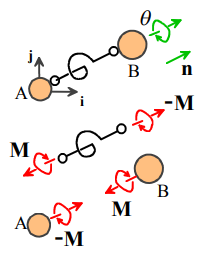The potential energy of the moments exerted by the spring can be determined by computing the work done to twist the spring through an angle θ .

1. The work done by a moment M due to twisting through a very small angle dθ about an axis parallel to a vector n is dW = M.dθn

2. The potential energy is the negative of the total work done by M, i.e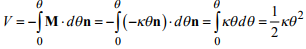4.1.6 Definition of the Kinetic Energy of a particle

Consider a particle with mass m which moves with velocity v = vxi + vyj + vzk. By definition, its kinetic energy is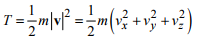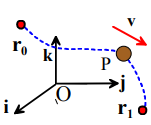4.1.7 Power-Work-kinetic energy relations for a single particle

Consider a particle with mass m that moves under the action of a force F. Suppose that

1. At some time t0 the particle has some initial position r0 , velocity v0 and kinetic energy T0
2. At some later time t the particle has a new position r, velocity v and kinetic energy T .
3. Let P = F. v denote the rate of work done by the force

4.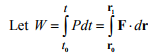be the total work done by the force

The Power-kinetic energy relation for the particle states that the rate of work done by F is equal to the rate of change of kinetic energy of the particle, i.e.

P = dT/dt

This is just another way of writing Newton’s law for the particle: to see this, note that we can take the dot product of both sides of F=ma with the particle velocity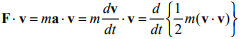To see the last step, do the derivative using the Chain rule and note that a.b = b. a.

The Work-kinetic energy relation for a particle says that the total work done by the force F on the particle is equal to the change in the kinetic energy of the particle.

W = T - T0

This follows by integrating the power-kinetic energy relation with respect to time.

4.1.8 Examples of simple calculations using work-power-kinetic energy relations

There are two main applications of the work-power-kinetic energy relations. You can use them to calculate the distance over which a force must act in order to produce a given change in velocity. You can also use them to estimate the energy required to make a particle move in a particular way, or the amount of energy that can be extracted from a collection of moving particles (e.g. using a wind turbine)

Example 1: Estimate the minimum distance required for a 14 wheeler that travels at the RI speed-limit to brake to a standstill. Is the distance to stop any different for a Toyota Echo?

This problem can be solved by noting that, since we know the initial and final speed of the vehicle, we can calculate the change in kinetic energy as the vehicle stops. The change in kinetic energy must equal the work done by the forces acting on the vehicle – which depends on the distance slid. Here are the details of the calculation.

Assumptions: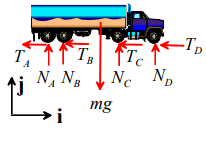1. We assume that all the wheels are locked and skid over the ground (this will stop the vehicle in the shortest possible distance)
2. The contacts are assumed to have friction coefficient μ = 0.8
3. The vehicle is idealized as a particle.
4. Air resistance will be neglected.

Calculation:

1. The figure shows a free body diagram.
2. The equation of motion for the vehicle is

- (TA +TB +TC +TD) i + (NA + NB + NC + ND - mg)j = maxi

The vertical component of the equation shows that  NA + NB + NC + ND = mg.
3. The friction force follows as (TA + TB + TC + TD) = μ (NA + NB + NC + ND) = μ mg
4. If the vehicle skids for a distance d, the total work done by the forces acting on the vehicle is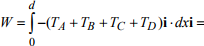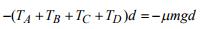5. The work-energy relation states that the total work done on the particle is equal to its change in kinetic energy. When the brakes are applied the vehicle is traveling at the speed limit, with speed V; at the end of the skid its speed is zero. The change in kinetic energy is therefore ΔT = 0 - mV2 /2. The work-energy relation shows that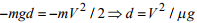Substituting numbers gives

This simple calculation suggests that the braking distance for a vehicle depends only on its speed and the friction coefficient between wheels and tires. This is unlikely to vary much from one vehicle to another. In practice there may be more variation between vehicles than this estimate suggests, partly because factors like air resistance and aerodynamic lift forces will influence the results, and also because vehicles usually don’t skid during an emergency stop (if they do, the driver loses control) – the nature of the braking system therefore also may change the prediction.

Example 3: Compare the power consumption of a Ford Excursion to that of a Chevy Cobalt during stopstart driving in a traffic jam.

During stop-start driving, the vehicle must be repeatedly accelerated to some (low) velocity; and then braked to a stop. Power is expended to accelerate the vehicle; this power is dissipated as heat in the brakes during braking. To calculate the energy consumption, we must estimate the energy required to accelerate the vehicle to its maximum speed, and estimate the frequency of this event.

Calculation/Assumptions:

1. We assume that the speed in a traffic jam is low enough that air resistance can be neglected.
2. The energy to accelerate to speed V is mV2 / 2 .
3. We assume that the vehicle accelerates and brakes with constant acceleration – if so, its average speed is V/2.
4. If the vehicle travels a distance d between stops, the time between two stops is 2d/V.
5. The average power is therefore mV d / 4 .
6. Taking V=15mph (7m/s) and d=200ft (61m) are reasonable values – the power is therefore 0.03m, with m in kg. A Ford Excursion weighs 9200 lb (4170 kg), requiring 125 Watts (about that of a light bulb) to keep moving. A Chevy Cobalt weighs 2681lb (1216kg) and requires only 36 Watts – a very substantial energy saving.

Reducing vehicle weight is the most effective way of improving fuel efficiency during slow driving, and also reduces manufacturing costs and material requirements. Another, more costly, approach is to use a system that can recover the energy during braking – this is the main reason that hybrid vehicles like the Prius have better fuel economy than conventional vehicles.

Example 4: Estimate the power that can be generated by a wind turbine.

The figure shows a wind turbine. The turbine blades deflect the air flowing past them: this changes the air speed and so exerts a force on the blades. If the blades move, the force exerted by the air on the blades does work – this work is the power generated by the turbine. The rate of work done by the air on the blades must equal the change in kinetic energy of the air as it flows past the blades. Consequently, we can estimate the power generated by the turbine by calculating the change in kinetic energy of the air flowing through it.

To do this properly needs a very sophisticated analysis of the air flow around the turbine. However, we can get a rather crude estimate of the power by assuming that the turbine is able to extract all the energy from the air that flows through the circular area swept by the blades.

Calculation: Let V denote the wind speed, and let p denote the density of the air.

1. In a time t, a cylindrical region of air with radius R and height Vt passes through the fan.
2. The cylindrical region has mass πR2pVt

3. The kinetic energy of the cylindrical region of air is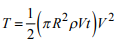4. The rate of flow of kinetic energy through the fan is therefore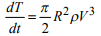5. If all this energy could be used to do work on the fan blades the power generated would be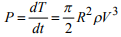Representative numbers are (i) Air density 1.2 kg m/3 ; (ii) air speed 25mph (11 m/sec); (iii) Radius 30m This gives 1.8MW. For comparison, a nuclear power plant generates about 500-1000 MW.

A more sophisticated calculation (which will be covered in EN810) shows that in practice the maximum possible amount of energy that can be extracted from the air is about 60% of this estimate. On average, a typical household uses about a kW of energy; so a single turbine could provide enough power for about 5- 10 houses.

4.1.9 Energy relations for a conservative system of particles.

The figure shows a `system of particles’ – this is just a collection of objects that we might be interested in, which can be idealized as particles. Each particle in the system can experience forces applied by: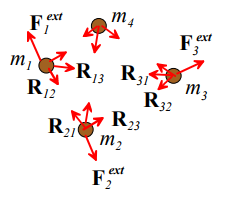Other particles in the system (e.g. due to gravity, electric charges on the particles, or because the particles are physically connected through springs, or because the particles collide). We call these internal forces acting in the system. We will denote the internal force exerted by the ith particle on the jth particle by Rij . Note that, because every action has an equal and opposite reaction, the force exerted on the jth particle by the ith particle must be equal and opposite, to Rij , i.e. Rij = -Rji.

Forces exerted on the particles by the outside world (e.g. by externally applied gravitational or electromagnetic fields, or because the particles are connected to the outside world through mechanical linkages or springs). We call these external forces acting on the system, and we will denote the on the i th particle by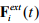We define the total external work done on the system during a time interval t0 < t < t0 + Δt as the sum of the work done by the external forces.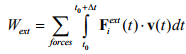The total work done can also include a contribution from external moments acting on the system. The system of particles is conservative if all the internal forces in the system are conservative. This means that the particles must interact through conservative forces such as gravity, springs, electrostatic forces, and so on. The particles can also be connected by rigid links, or touch one another, but contacts between particles must be frictionless.

If this is the case, we can define the total potential energy of the system as the sum of potential energies of all the internal forces.

We also define the total kinetic energy Ttotal of the system as the sum of kinetic energies of all the particles.

The work-energy relation for the system of particles can then be stated as follows. Suppose that

1. At some time t0 the system has and kinetic energy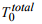2. At some later time t the system has kinetic energy Ttotal .

3. Let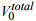denote the potential energy of the force at time t0

4. Let Vtotal denote the potential energy of the force at time t

5. Let Wext denote the total work done on the system between t0 < t < t0 + Δt

Work Energy Relation: This law states that the external work done on the system is equal to the change in total kinetic and potential energy of the system.

Wext = T + V - (T0 + V0)

We won’t attempt to prove this result - the proof is conceptually very straightforward: it simply involves summing the work-energy relation for all the particles in the system; and we’ve already seen that the work-energy relations are simply a different way of writing Newton’s laws. But when written out the sums make the proof look scary and difficult to follow so we’ll spare you the gory details. If you are interested, ask us (or better still see if you can do it for yourself!)

Energy conservation law For the special case where no external forces act on the system, the total energy of the system is constant

Wext = 0⇔T + V = (T0 + V0)

It is worth making one final remark before we turn to applications of these law. We often invoke the principle of conservation of energy when analyzing the motion of an object that is subjected to the earth’s gravitational field. For example, the first problem we solve in the next section involves the motion of a projectile launched from the earth’s surface. We usually glibly say that `the sum of the potential and kinetic energies of the particle are constant’ – and if you’ve done physics courses you’ve probably used this kind of thinking. It is not really correct, although it leads to a more or less correct solution.

Properly, we should consider the earth and the projectile together as a conservative system. This means we must include the kinetic energy of the earth in the calculation, which changes by a small, but finite, amount due to gravitational interaction with the projectile. Fortunately, the principle of conservation of linear momentum (to be covered later) can be used to show that the change in kinetic energy of the earth is negligibly small compared to that of the particle.

Offer running on EduRev: Apply code STAYHOME200 to get INR 200 off on our premium plan EduRev Infinity!

## Introduction to Dynamics and Vibrations- Notes, Videos, MCQs

20 videos|53 docs

,

,

,

,

,

,

,

,

,

,

,

,

,

,

,

,

,

,

,

,

,

,

,

,

,

,

,

;